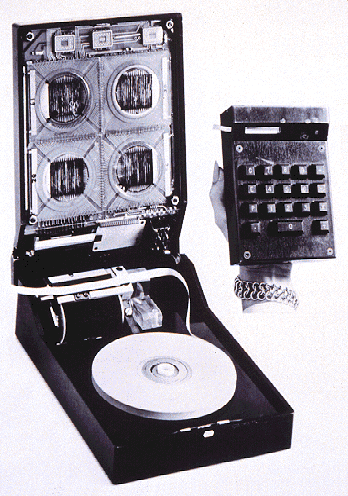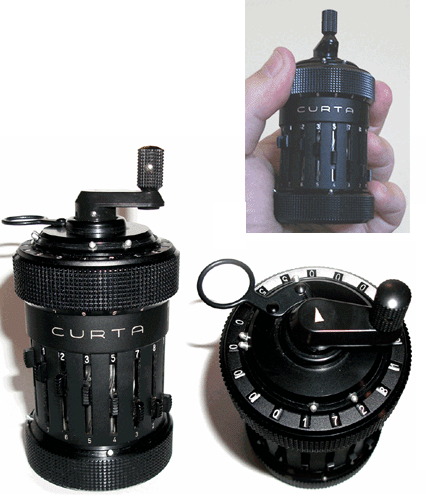# calculator

(redirected from calculate)
Also found in: Dictionary, Thesaurus, Medical, Acronyms, Idioms.

## calculator

calculator or calculating machine, device for performing numerical computations; it may be mechanical, electromechanical, or electronic. The electronic computer is also a calculator but performs other functions as well.

### Mechanical and Electromechanical Calculators

Early devices used to aid in calculation include the abacus (still common in E Asia) and the counting rods, or “bones,” of the Scottish mathematician John Napier. The slide rule, invented in 1622 by William Oughtred, an English mathematician, was widely used to make approximate calculations, but it has been replaced by the electronic calculator. In 1642, Blaise Pascal devised what was probably the first simple adding machine using geared wheels.

In 1671 an improved mechanism for performing multiplication by the process of repeated addition was designed by Gottfried W. von Leibniz. A machine using the Leibniz mechanism was the first to be produced successfully on a commercial scale; devised in 1820 by the Frenchman Charles X. Thomas, it could be used for adding, subtracting, multiplying, or dividing. A mechanism permitting the construction of a more compact machine than the Leibniz mechanism was incorporated into a machine devised late in the 19th cent. by the American inventor Frank S. Baldwin. Later the machine was redesigned by Baldwin and another American inventor, Jay R. Monroe. At about the same time, W. T. Odhner of Russia constructed a machine using the same device as Baldwin's. Charles Babbage, an English mathematician, and William S. Burroughs, an American inventor, also made important contributions to the development of the calculating machine.

Early mechanical adding machines were equipped with a keyboard on which numbers to be added were entered, a lever to actuate the addition process, and an accumulator to display the results. A full keyboard consisted of 10 columns of keys with 9 keys in each column, numbered 1 through 9. Each column could be used to enter a figure in a particular decimal place so that a number up to 10 digits long could be entered; if no key was pressed in a given column, a zero was entered in that decimal place. The lever was pulled in one direction when a number was to be added and in the opposite direction when it was to be subtracted. The accumulator was a set of geared wheels, each corresponding to a decimal place and having the digits 0 through 9 printed on its circumference. When a given wheel made a complete rotation, the next wheel was advanced by one digit. The mechanical adding machine remained essentially the same until the mid-1960s, with improvements consisting of motors to actuate additions and subtractions and mechanisms to print out results on a paper tape.

### Electronic Calculators

Electronic calculators, which became available in the early 1960s, at first were merely faster and quieter desktop adding machines. The invention of the microprocessor and advances in integrated-circuit technology made small, but highly sophisticated, hand-held calculators possible, and by the mid-1970s they were in wide use. Simple calculators perform only the basic four functions of addition, subtraction, multiplication, and division. More sophisticated calculators can perform trigonometric, statistical, logarithmic, and other advanced calculations.

Some electronic calculators are actually small computers with limited memory and programming capabilities. Some of these programmable calculators can accept plug-in semiconductor memory cards or programming modules for special applications, such as financial calculations, unit, currency, or number-system conversions, or engineering calculations. Others are also available that include nonmathematical functions such as data storage and schedule organizing. The personal digital assistant, a hand-held device optimized as an organizer with communications capability and accepting handwritten input, was a bridge from calculators to full computer function.

Early electronic calculators had numeric displays made from light-emitting diodes (LEDs). They have been supplanted by liquid-crystal displays (LCDs), whose lower power consumption helps to reduce battery drain. Some calculators use an LCD readout to provide a graphic, as well as numeric, display. CMOS, or complementary metal-oxide-semiconductor (see integrated circuit), technology is also preferred for battery-operated models because of its low-power requirements. Some calculators are powered by solar cells in ordinary room light.

### Bibliography

See B. Randell, The Origins of Digital Computers: Selected Papers (1982); J. P. Haney, Calculators (3d ed. 1985).

## calculator

[′kal·kyə‚lād·ər]
(computer science)
A device that performs logic and arithmetic digital operations based on numerical data which are entered by pressing numerical and control keys. Also known as calculating machine.
McGraw-Hill Dictionary of Scientific & Technical Terms, 6E, Copyright © 2003 by The McGraw-Hill Companies, Inc.

## calculator

1. a device for performing mathematical calculations, esp an electronic device that can be held in the hand
2. a set of tables used as an aid to calculations
Collins Discovery Encyclopedia, 1st edition © HarperCollins Publishers 2005

## calculator

A machine that provides arithmetic capabilities. The most basic calculators add, subtract, multiply and divide. More advanced devices handle sines, cosines and other trigonometric functions. Whether mechanical or electronic, all calculators accept numeric input from dials, levers or a keypad. The results are displayed as mechanical digits on paper tape or on a screen, such as an LCD. Unlike computers, calculators perform a finite set of arithmetic functions, and they cannot handle text. See Comptometer.

The First HandheldIn 1967, Texas Instruments introduced the first handheld calculator. The opened unit in the picture shows the paper tape used as output. (Image courtesy of Texas Instruments, Inc.)

The Curta Pepper GrinderCompleted in a World War II concentration camp in the 1940s, Curt Herzstark was given permission to perfect the calculator he patented earlier. These mechanical marvels were used up to the 1980s for their accuracy and ruggedness in hostile conditions such as car rallies. The sliders on the side hold the number multiplied by the number of times the "grinder" on top is turned. The results appear on top as well. (Image courtesy of Clive "Max" Maxfield, www.clivemaxfield.com)
Copyright © 1981-2019 by The Computer Language Company Inc. All Rights reserved. THIS DEFINITION IS FOR PERSONAL USE ONLY. All other reproduction is strictly prohibited without permission from the publisher.
References in periodicals archive ?
The system then analyzes the results, calculates modulus values and suggests the number and location of required risers.
A new algorithm for the GDC system imports speed data from encoders on the winder and nip rolls and calculates shrink or stretch based on the difference between winder and nip speeds.
More than a decade ago, researchers were surprised to learn that using the Boltzmann equation to calculate simple fluid flows didn't make the simulations more difficult or time consuming to carry out.
To calculate the power passing through the aperture A of vertical size [DELTA]Y and horizontal size [DELTA]X, positioned at distance d from the source point S, the result of the convolution in Eq.
Using this initial compound and molded product data, the HR-soft program calculates a cure time characteristic chart.
Once the appropriate background information has been entered, a user simply presses the "calculate" button.
POLYNOMIAL POWER A computer specially geared to calculate the value of some obscure knot invariant might not seem particularly useful.
The staff then plugs in the numbers to calculate several commensurate rent increase proposals that theoretically would allow owners to remain whole despite the price increases.
Riser Calculation: FeederCalc for Iron was used to calculate the riser.
The traditional way to measure sensitivity of an instrument is to calculate the signal-to-noistatic ratio.
The predictor program was originally developed by PMC for in-house use to calculate the mold tolerance for a particular part.
(If an issuer does not need to calculate the OID separately, only one amortization schedule is needed to determine the total deduction for interest and debt issuance costs, lf an issuer, however, must report OID to holders or must calculate OID to apply certain interest limits, it will have to construct both schedules, because debt issuance costs are not considered OID.)

Site: Follow: Share:
Open / Close